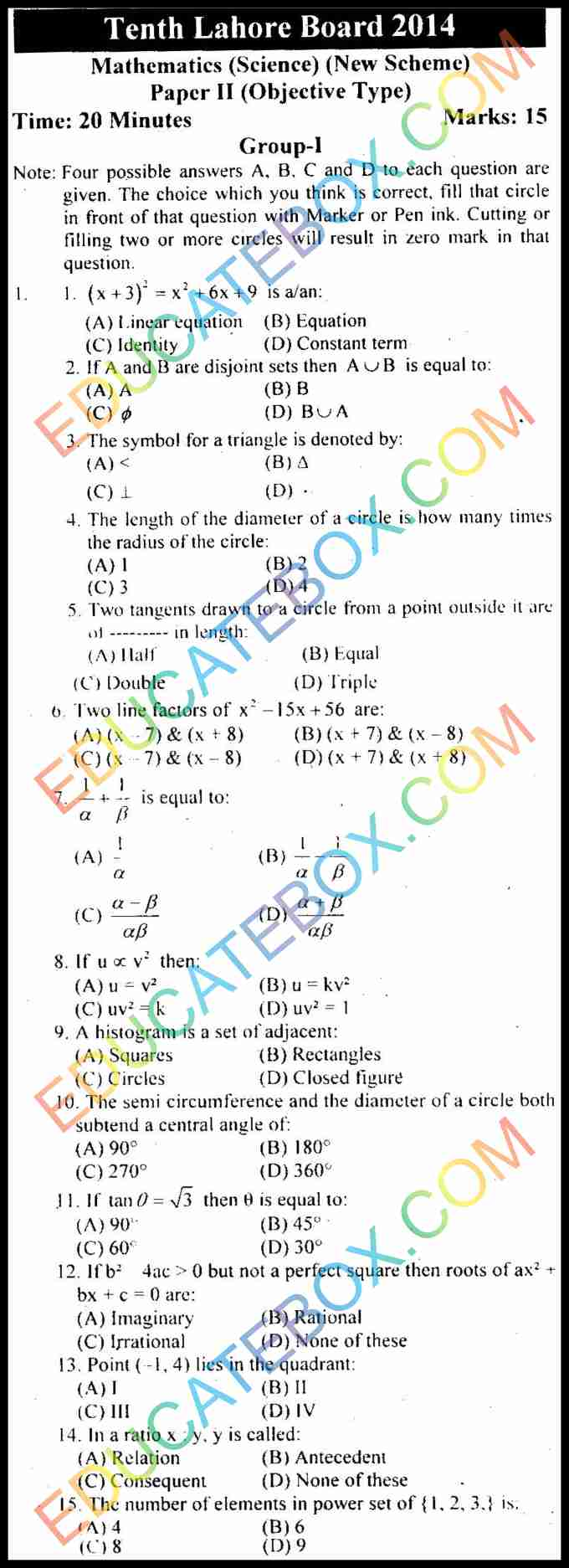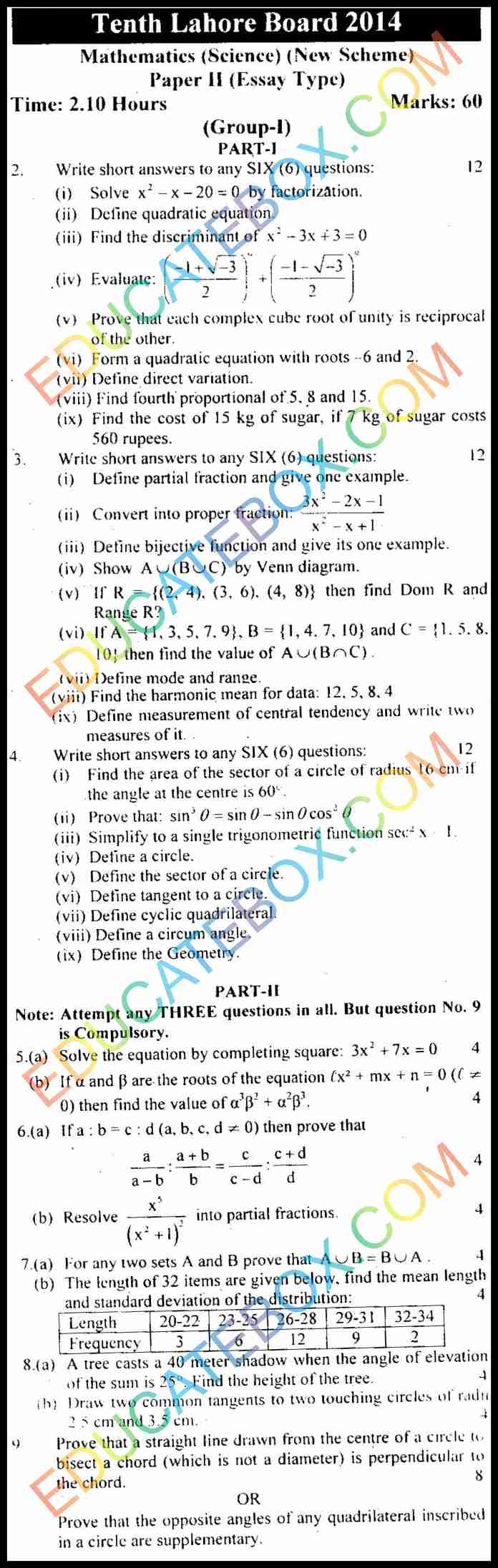# Past Paper 10th Maths (Science Group) Lahore 2014 Group 1

0
102

## Past Paper Class 10 Maths (Science Group) Lahore Board 2014 Objective Type Group 1 (English Medium)

Here is Maths (Science Group) 10th Lahore Board paper of 2014 Group 1.

In the first part, there are fourteen multiple choice questions. All the questions carry equal marks. There are four possible answers to each question. Cutting or filling two or more circles will result in zero mark in that question.

Some of the objective and subjective questions are as follows:

• What is symbol of triangle?
• The length of the diameter of a circle is how many times the radius of the circle.
• What is the length of two tangents drawn to a circle from a point outside it.
• What is the semi circumference and diameters of a circle both subtend a central angle.
• What should be the number of elements in power set of { 1, 2, 3,}?
• Prove that each complex cube root of unity is reciprocal of the other
• Find the cost of 15 kg of sugar, if 7 kg of sugar costs 560 rupees.
• Define measurement of central tendency and write two measures of it
• prove that the opposite angles of any quadrilateral inscribed in a circle## Past Paper Class 10 Maths (Science Group) Lahore Board 2014 Objective Type Group 1 (English Medium)